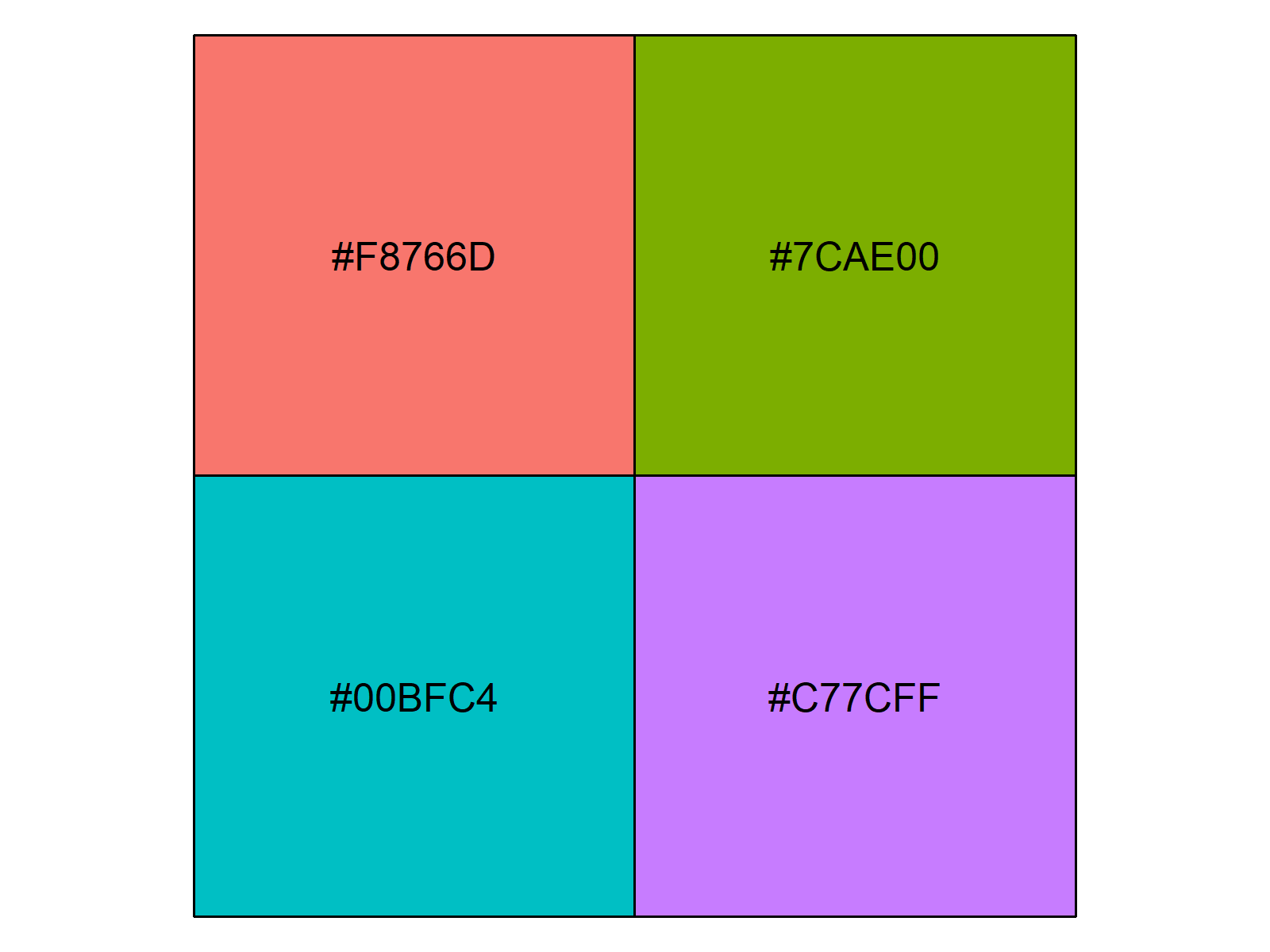# Extract Default Color Palette of ggplot2 R Package (Example)

This tutorial shows how to emulate the ggplot2 default color palette in the R programming language.

The content of the page looks as follows:

## Creation of Example Data

Let’s create some example data and a ggplot2 plot in order to illustrate how to identify the default color palette of the ggplot2 package.

First, we need to create an exemplifying data frame:

```data <- data.frame(group = LETTERS[1:4],                # Create example data
prob = c(2, 1, 5, 3))```

Furthermore, we need to install and load the ggplot2 package to R:

```install.packages("ggplot2")                             # Install ggplot2 R package
library("ggplot2")                                      # Load ggplot2 R package```

Now, we can create a barchart of our example data based on the ggplot2 package as follows:

```ggplot(data, aes(x = group, y = prob, fill = group)) +  # Create ggplot2 barchart
geom_bar(stat = "identity")```Figure 1: Basic Barchart Created with the ggplot2 R Package.

Figure 1 illustrates our example barchart. As you can see, the barchart contains four bars, each with a different color.

In the following example, I’ll show you how to identify the hex codes of these colors in R. So keep on reading!

## Example: Extract Default Color Palette of ggplot2

An easy way to extract the default colors of the ggplot2 package is provided by the scales R package. Let’s install and load the package:

```install.packages("scales")                              # Install scales R package
library("scales")                                       # Load scales R package```

Now, we can use the following R code to get the hex codes of our previous ggplot2 barplot:

```n1 <- nrow(data)                                        # Amount of default colors
hex_codes1 <- hue_pal()(n1)                             # Identify hex codes
hex_codes1                                              # Print hex codes to console
# "#F8766D" "#7CAE00" "#00BFC4" "#C77CFF"```

As you can see based on the previous output of the RStudio console, we saved the hex codes in the data object hex_codes1.

The scales package also provides a nice way to visualize the colors:

`show_col(hex_codes1)                                    # Plot hex codes/colors`Figure 2: Four Default Colors & Hex Codes of the ggplot2 Color Palette.

Figure 2 shows the default colors of the ggplot2 package and the hex codes in a nice graph.

Of cause, we could do the same procedure for ggplots with a different amount of colors. For instance, we could get the first 50 default colors as shown below:

```n2 <- 50                                                # Higher amount of hex colors
hex_codes2 <- hue_pal()(n2)                             # Identify hex codes
show_col(hex_codes2)                                    # Plot hex codes/colors```Figure 3: Fifty Default Colors & Hex Codes of the ggplot2 Color Palette.

## Video & Further Resources

Have a look at the following video of my YouTube channel. I illustrate the R codes of this tutorial in the video:

Please accept YouTube cookies to play this video. By accepting you will be accessing content from YouTube, a service provided by an external third party.If you accept this notice, your choice will be saved and the page will refresh.

Furthermore, you could have a look at the other articles of this website.

Summary: On this page, I explained how to return the default ggplot2 colors based on the scales package in R programming. If you have any further questions, let me know in the comments section.

Subscribe to the Statistics Globe Newsletter